Charting Examples

For each of the chart types their data format expected together with some examples are shown below

Time Series

Format

1. The first date/time column found will be used for the x-axis.
2. Each numerical column represents one time series line on the chart.

Day Sines

A sine/cosine wave over a period of days.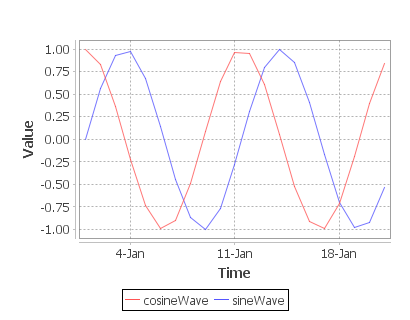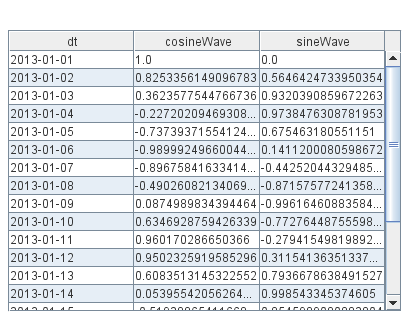Kdb Code

Position and Costs throughout the day.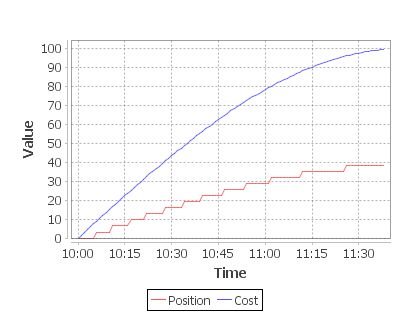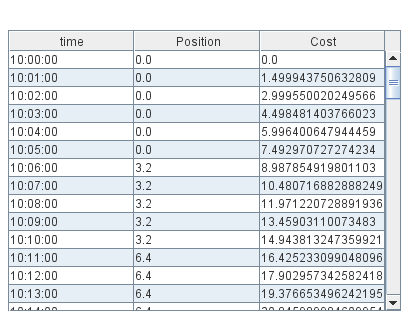Kdb Code

Line Chart

Format

1. The first string columns are used as category labels.
2. Whatever numeric columns appear after the strings represents a separate series in the chart.

Multiple Series with Time X-Axis

2 series are shown, one for Sales, One for Costs. x-axis is in months.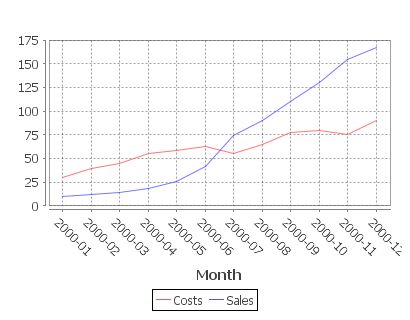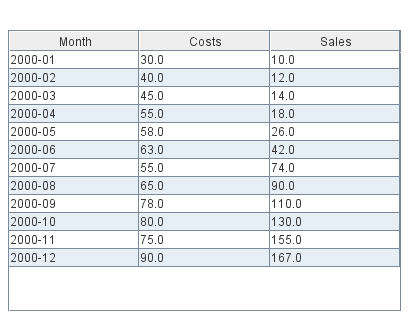Kdb Code

Single Category

One number column generates one series.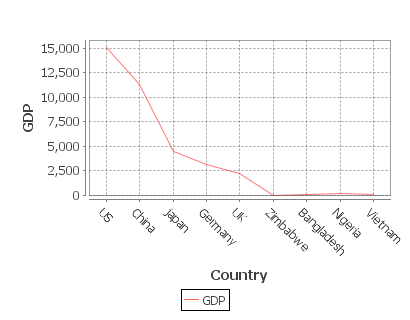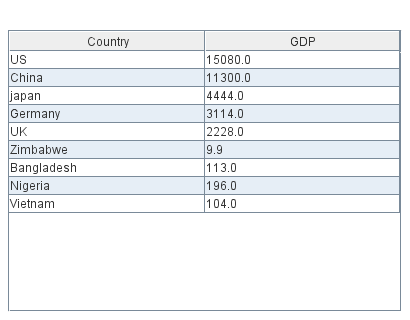Kdb Code

Multiple series

Each column is a new series/colored bar.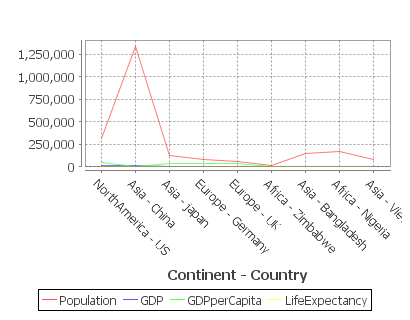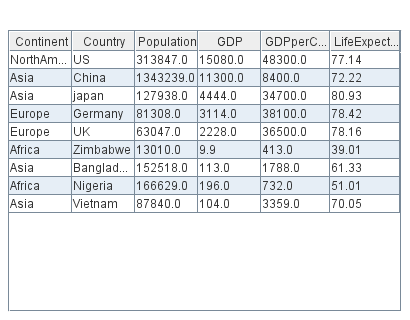Kdb Code

Area Chart

Format

1. The first string columns are used as category labels.
2. Whatever numeric columns appear after the strings represents a separate series in the chart.

Multiple Series with Time X-Axis

2 series are shown, one for Sales, One for Costs. x-axis is in months.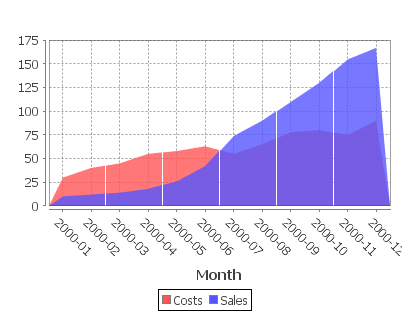Kdb Code

Single Category

One number column generates one series.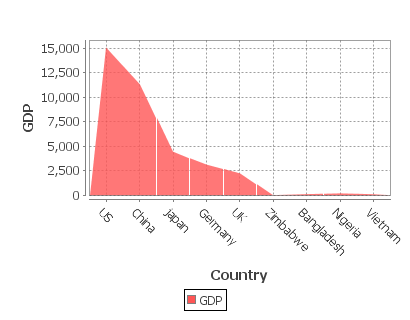Kdb Code

Multiple series

Each column is a new series/colored bar.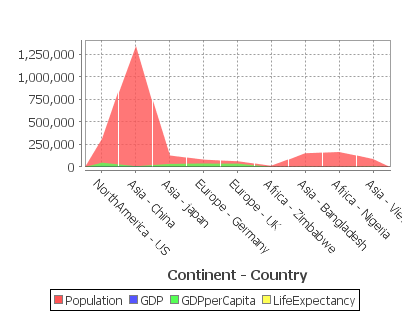Kdb Code

Bar Chart

Format

1. The first string columns are used as category labels.
2. Whatever numeric columns appear after the strings represents a separate series in the chart.

Multiple Series with Time X-Axis

2 series are shown, one for Sales, One for Costs. x-axis is in months.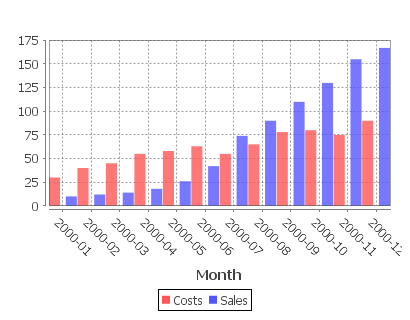Kdb Code

Single Category

One number column generates one series.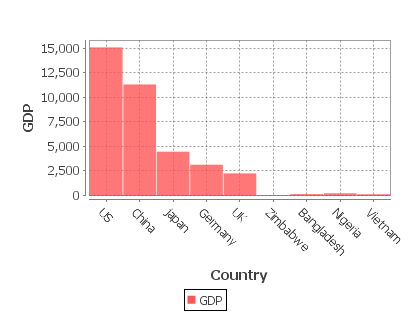Kdb Code

Multiple series

Each column is a new series/colored bar.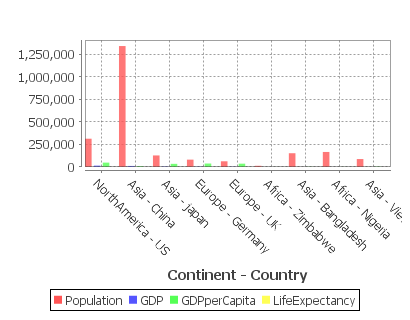Kdb Code

Bar Chart Stacked

Format

1. The first string columns are used as category labels.
2. Whatever numeric columns appear after the strings represents a separate series in the chart.

Multiple Series with Time X-Axis

2 series are shown, one for Sales, One for Costs. x-axis is in months.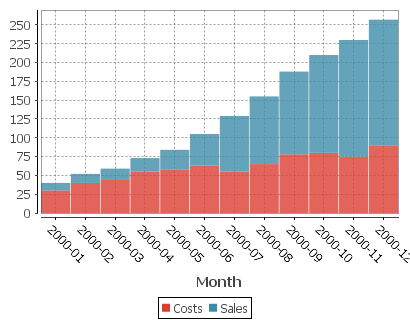KDB Code

Multiple series

Each column is a new series/colored bar.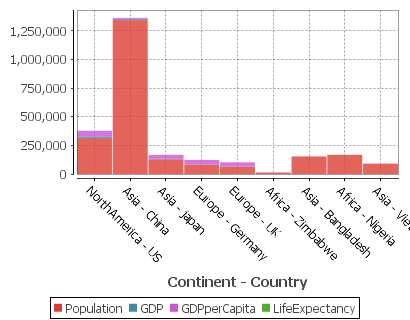KDB Code

Multiple series with many categories on the X-axis

Each column is a new series/colored bar. When there are a lot of categories, some labels are hidden.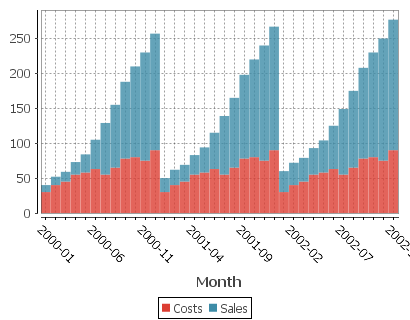KDB Code

Bubble Chart

Format

1. The first string columns are used as category labels.
2. There must then be 3 numeric columns which are used for x-coord, y-coord, size in that order.

Single series

The three columns are used for x-axis,y-axis and size respectively. Notice the GdpPerCapita column has been divided to make it similar sized to the othercolumns so that the bubbles are a sensible size.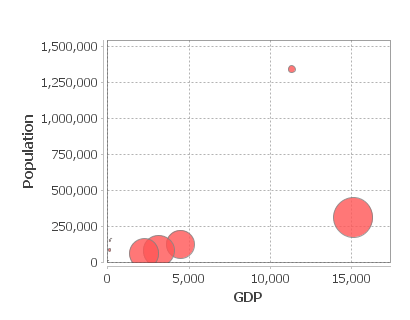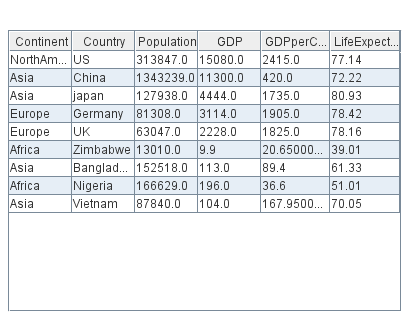Kdb Code

Candlestick

Format

The table should contain columns labelled open/high/low/close/volume
but must atleast contain high/low to allow it to be drawn.

Prices going up

A Candlestick showing decreasing prices after 9AM but increasing volumes of trade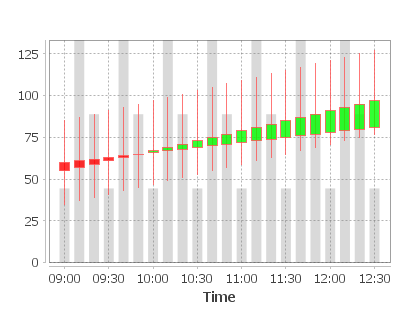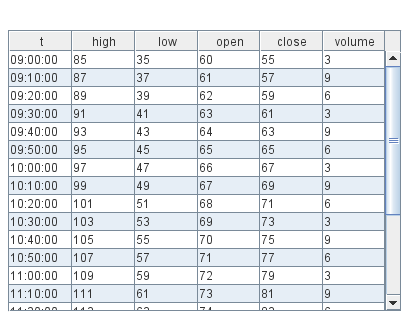Kdb Code

Data Table

Format

Any format of table is acceptable, all rows/columns will be shown as a plain table.

Many Columned Table

All rows/columns will be shown as a plain table.Kdb Code

Heat Map

Format

A HeatMap works best with 1+ string columns.

• Starting from the left each string column is taken as one nesting level
• The first numerical column will be taken as size, the second as colour.

Country GDP's

The continent column is a top-level branch, the country column becomes leafs.The first two columns are GDP and GDP per Capita which become the size and color of the leafs respectively.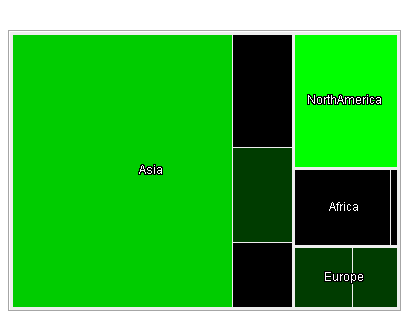Kdb Code

Histogram

Format

Each Numeric column represents a separate series in the histogram. The series values are placed into buckets and their frquency tallied.

Profit Distribution

Distribution of Returns and Losses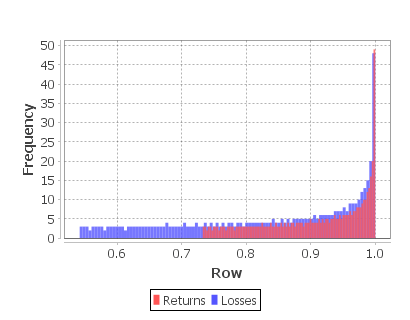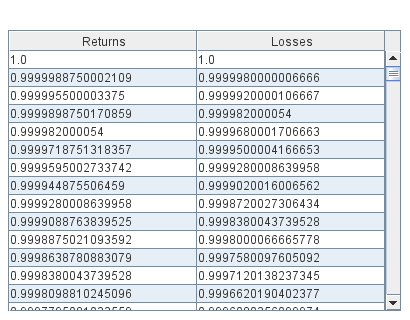Kdb Code

Pie Chart

Format

Each numerical column represents a pie chart.The title of each pie chart will be the column title.Each row will be a section of the pie and will use the row title as a label.

Single Pie Chart Example

A single numerical columns gives a single pie chart for that column.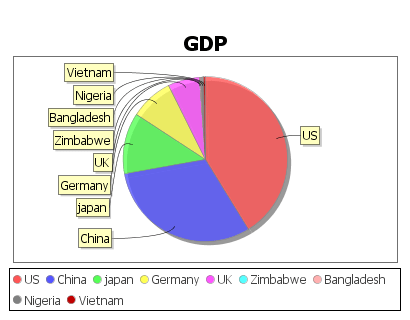Kdb Code

Multiple Pie Chart Example

Using multiple numerical columns gives a pie chart for each column.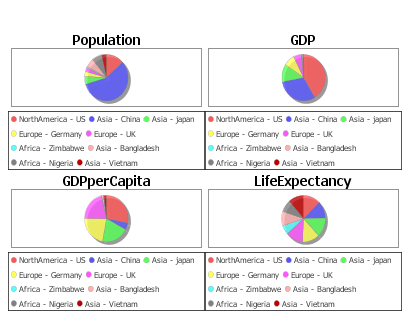Kdb Code

Scatter Plot

Format

• Two or more numeric columns are required.
• The values in the first column are used for the X-axis.
• The values in following columns are used for the Y-axis.
• Each column is displayed with a separate color.

Country Population and GDP

The first column GDP is used for the x-axis. The subsequent columns are then plotted against that x axis as separate colored series.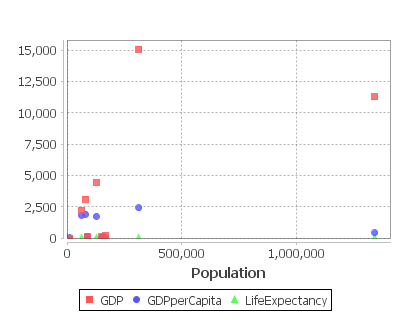Kdb Code

Step Plot

Format

1. The first date/time column found will be used for the x-axis.
2. Each numerical column represents one time series line on the chart.

Day Sines

A sine/cosine wave over a period of days.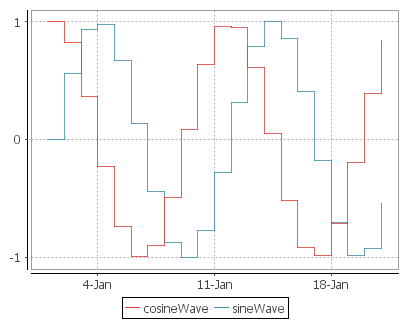KDB Code

DOT Graph

Format

1. The table can contain columns labelled from/to/label/style.
2. If it does not, the first two columns are assumed to be from and to respectively.
3. The dot file generated gives a directed graph from "from" nodes to "to" nodes with applicable style/labels.
4. Graphviz Dot MUST be on your command line PATH for this to work.

Alphabet Spaghetti

A directed graph with 5 nodes.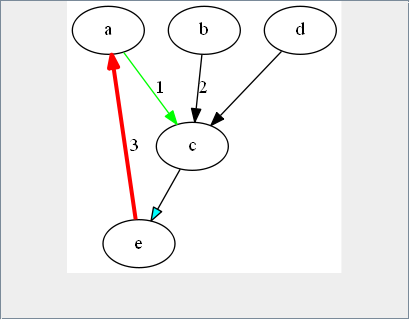KDB Code